CS 696: Advanced OO Spring Semester, 1997 Doc 8, Metrics part 2

To Lecture Notes Index
San Diego State University -- This page last updated Feb 25, 1997Contents of Doc 8, Metrics part 2

 McCabe's Cyclomatic Complexity slide # 2 Henderson-Sellers' Recommended OO Metric Suite slide # 4 ...Inside a class slide # 4 ...Metrics External at the Class level slide # 6 ...System Level but Ignoring Relationships - Metrics slide # 7 ...System-Level Relationships but Excluding Inheritance slide # 8 ...Inheritance Coupling slide # 9

Doc 8, Metrics part 2 Slide # 1
References

Henderson-Sellers, 1996, Object-Oriented Metrics: Measures of Complexity, Prentice Hall

Doc 8, Metrics part 2 Slide # 2

McCabe's Cyclomatic Complexity

Logic Structure Metric

Let G be a connected, directed acyclic graph (DAG) with e edges and n nodes

The cyclomatic complexity (or cycle rank) of G is defined as:

V(G) = e - n + 2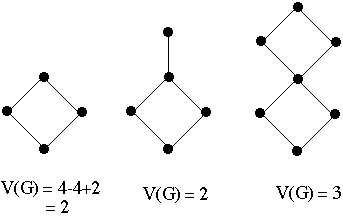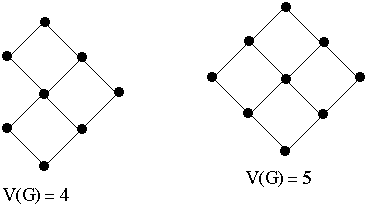Doc 8, Metrics part 2 Slide # 3
Cyclomatic Complexity and Code Complexity

 if ( A< B ) then W; else X; endif A = 3;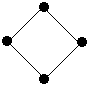V(G) = 2 if ( A< B ) then W; else if ( B < C ) then X; else Z; endif endif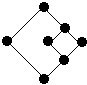V(G) = 3 switch( A ) case 1: X; break; case 2: Y; break; case 3: Z; break; end case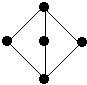V(G) = 3

Doc 8, Metrics part 2 Slide # 4

Henderson-Sellers' Recommended OO Metric Suite

Inside a class

Assume that a class has:
n public methods
m public data members
r private methods
s private data members

Control Flow Complexity

For each class compute:

• V(G) for each of the n + r methods
• A distribution of the n + r values of V(G)
• A median of the n + r values of V(G)
• A mean value of the n + r values of V(G)
• A standard deviation of the n + r values of V(G)
• A class total V(G)
Note the class total for V(G) is one interpretation of WMC

Doc 8, Metrics part 2 Slide # 5
Inside a class - Metrics

Method Size

For each class compute:

• Size of each method (SLOC)
• A distribution of the method sizes of a class
• A median of the method sizes of a class
• A mean value of the method sizes of a class
• A standard deviation of the method sizes of a class
• A class total method size (TMS)

Class Size

• A class total number of all methods (NOM)
• A class total number of attributes (data members) (NOA)
• Weighted class size = NOA + TMS
• Unweighted class size = NOA + NOM

Other Class Metrics

• Number of preconditions
• Number of postconditions
• Lack of Cohesion in Methods (LCOM*)
• Number of friend classes

Note the ratios preconditions/method and postconditions/method should tend to unity

Doc 8, Metrics part 2 Slide # 6

Metrics External at the Class level

Queries are methods that return a value
Commands are methods that don't return a value

• Number of commands for k'th class, ck
• Number of queries for k'th class, qk

• Number of weighted commands for k'th class, c'k
• Number of weighted queries for k'th class, q'k

Let Args = total number of arguments in all methods of a class
Then

c'k = ck + Args
q'k = qk+ Args

Doc 8, Metrics part 2 Slide # 7

System Level but Ignoring Relationships - Metrics

• Total number of classes in system, Cs
• A mean value for the number of commands per class across the system, given as: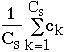• A standard deviation for ck
• A mean value for the number of queries per class across the system
• A standard deviation for qk
• A mean value for the number of weighted commands per class across the system,
• A standard deviation for c'k
• A mean value for the number of weighted queries per class across the system
• A standard deviation for q'k
• Average class size
• Standard deviation for class sizes
• Distribution of class sizes
• Average number of methods per class• Standard deviation for NOMk
• Distribution of NOMk

System-Level Relationships but Excluding Inheritance

• Coupling Between Objects (CBO) = fan-out
"A useful insight into the 'object-orientedness' of the design can be gained from the system wide distribution of the class fan-out values. For example a system in which a single class has very high fan-out and all other classes have low or zero fan-outs, we really have a structured, not an OO , system"

• Response for a Class RFC = NLM + NRM

NLM = number of local methods in the class
NRM = number of remote methods called by methods in the class

• Message-Passing Coupling (MPC)
number of send statements defined in a class

Doc 8, Metrics part 2 Slide # 9

Inheritance Coupling

• Depth of Inheritance Tree (DIT) for class
• Max depth of inheritance tree
• Mean depth of inheritance tree
• NOCk = number of children for each class
• Average NOC for the whole inheritance tree
• Standard distribution of NOC for the whole inheritance tree
• Distribution of NOC for the whole inheritance tree
• Reuse Ratio, U
• Specialization Ratio, S
where:
U = (number of superclasses)/ total number of classes

S = (number of subclasses)/ (number of superclasses)

Extreme Values of U and S
 Deep Hierarchy Wide Hierarchy U 1- 0 S 1+ infinity